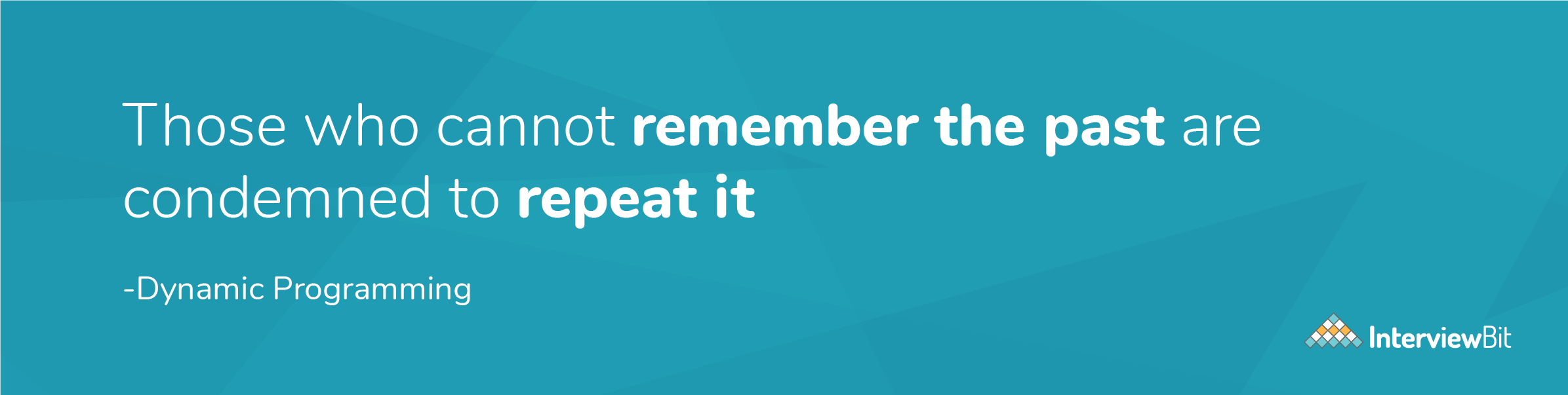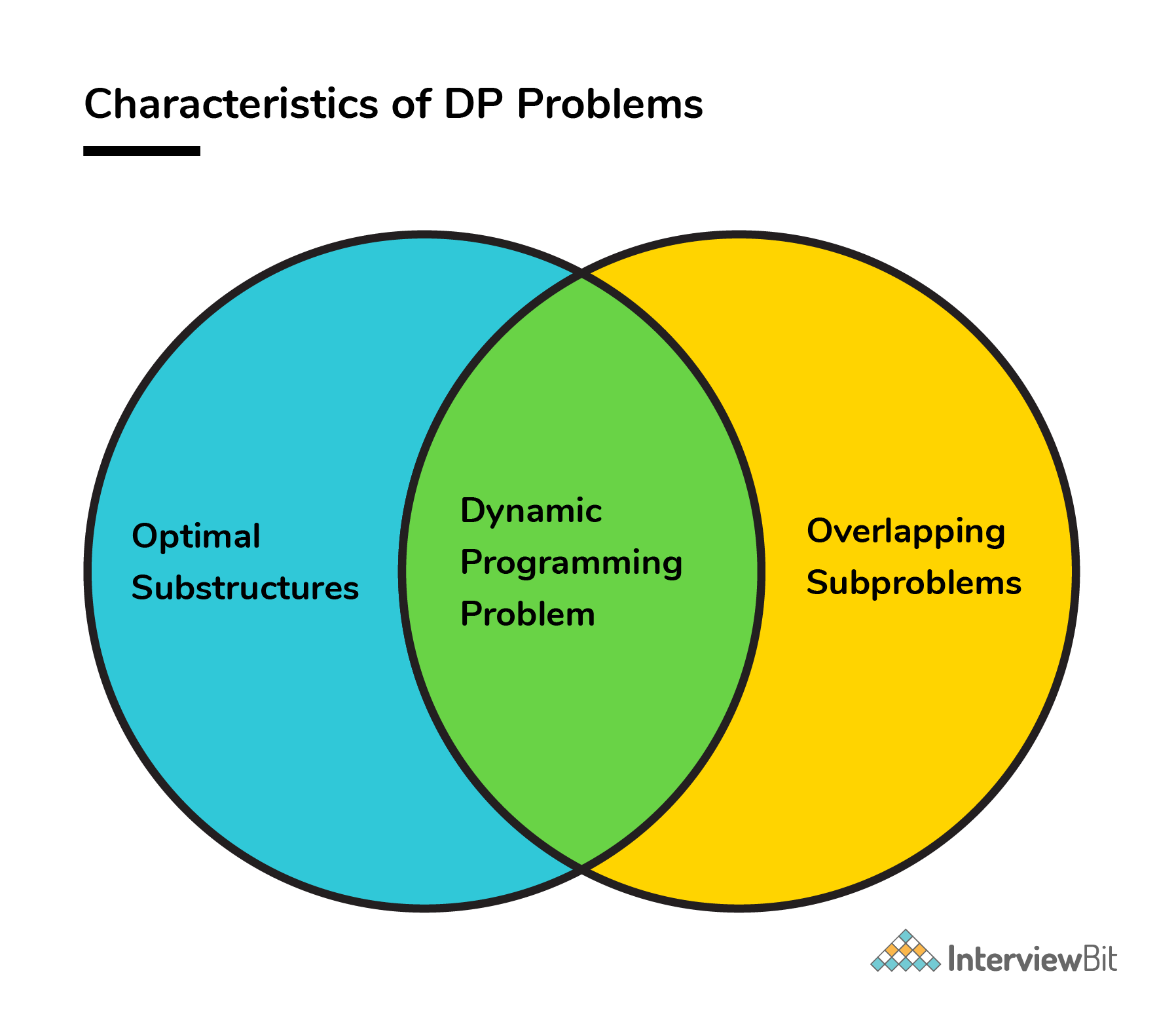Practice
Resources
Contests
Online IDE
New
Free Mock
Scaler
Practice
Improve your coding skills with our resources
Contests
Compete in popular contests with top coders
Scaler
Explore Offerings by SCALER## Welcome to Interviewbit, help us create the best experience for you!

Currently, You are a:College/University *
Enter the name of your college
Branch *
Year of completion *College/University *
Enter the name of your college
Branch *
Year of completion *Current Company *
Enter company name
Experience *## You're all set!Full name *
Email *

By creating an account, I acknowledge that I have read and agree to InterviewBit’s Terms and Privacy Policy .## Welcome back!

Email *Go to Problems

Be a Code Ninja!

# FAQs

### 1. Why is dynamic programming named “dynamic”?

• According to Richard Bellman’s autobiography “Eye of the Hurricane: An Autobiography (1984)”, the word “dynamic” was chosen by him to mainly capture the time-varying aspect of the problems.

### 2. How to recognize a problem that can be solved using Dynamic Programming?

• DP is mainly an optimization technique. It is a method for solving problems by breaking them down into simpler subproblems and solving and storing the results of each subproblem just once. If the same subproblem occurs again, we look up the previously stored solution.
• Hence, to recognize a problem and whether it can be solved using DP, ask yourself whether the given problem solution can be expressed as a function of solutions to similar smaller subproblems.

### 3. How to solve dynamic programming problems?

• The concept of dynamic programming is very simple. If we have solved a problem with the given input, then we save the result for future reference, so as to avoid recomputing again.

• If a given problem can be broken up in to smaller subproblems and these smaller subproblems can be in turn broken down in to even more smaller ones, and in this process, if we observe some subproblems which are already solved, then this is a big hint for us to use DP.
• In case we are not storing the results, then we are bound to perform computations unnecessarily which goes against the principle of dynamic programming.Image Source: Google
• We need to know that the optimal solutions to each subproblem contribute to the optimal solution of the overall given problem.

• We can follow the below steps as a guideline for coming up with a DP solution:

``````Step 1. Think of a recursive approach to solving the problem which essentially expresses a problem, say P(X), in terms of smaller subproblem, say P(Y) or an expression involving multiple smaller subproblems, say P(Yi). Here we expect Yi < X which could mean one of the following:
a. If X is an integer, then it could mean Yi "less than" X arithmetically.
b. If X is a string, it could mean that Yi is a substring of X.
c. If X is an array, it could mean Yi is a subarray of X, and so forth.

Step 2. Once you have a approach, write a recursive code for that. Consider your recursive code function definition to be as below :
solve(K1, K2, K3 ... )
Step 3. Keep track of the results of each function by saving them after every function call so that if the same function solve(K1, K2, K3 ... ) is called again, we need not compute again.

Step 4. Once we are done, analyze the space and time complexities of the solution developed, and try to improve them if possible.

``````

And that’s how a DP problem is solved.

### 4. Difference between the top-down approach (memoization) and the bottom-up approach (tabulation)?

Parameters Memoization Tabulation
State definition State definition can be thought of easily. Complicated to identify what a state should represent
Ease of code Less complicated and easy to code. Complications increase when lots of other conditions arise.
Speed of execution Slower due to recursive calls and return statements Faster as state values are accessed directly from table.
Space The cache entries are filled on demand during memoization. All entries starting from the first one needs to be filled by default.

### 5. Where is dynamic programming used?

• Dynamic programming is used in the cases where we solve problems by dividing them into similar suproblems and then solving and storing their results so that results are re-used later.
• Used in the cases where optimization is needed.
• Please refer to Application section above.

### 6. What are the characteristics of dynamic programming?

• Every DP problem should have optimal substructure and overlapping subproblems.• Please refer to the Characteristics of Dynamic Programming section above.

### 7. What are the applications of dynamic programming?

• DP is almost used everywhere which requires a guaranteed optimal solution. It is heavily used in routing, graph problems, computer vision, computer networks, AI, machine learning etc.
• Please refer to the Application section above.

### 8. How is dynamic programming different from the Greedy approach?

Greedy Algorithm vs Dynamic Programming

Parameters Dynamic Programming Greedy Approach
Optimality There is guaranteed optimal solution as DP considers all possible cases and then choose the best among them. Provides no guarantee of getting optimum approach.
Memory DP requires a table or cache for remembering and this increases it’s memory complexity. More memory efficient as it never looks back or revises its previous choices.
Time complexity DP is generally slower due to considering all possible cases and then choosing the best among them. Generally faster.
Feasibility Decision at each step is made after evaluating current problem and solution to previously solved subproblem to calculate optimal solution. Choice is made which seems best at the moment in the hope of getting global optimal solution.

### 9. How is dynamic programming different from the divide and conquer approach?

• Divide and Conquer algorithm works by dividing a problem into subproblems, conquer by solving each subproblem recursively and then combine these solutions to get solution of the main problem.
• Whereas DP is a optimization technique for solving problems in an optimised manner by dividing problem into smaller subproblems and then evaluating and storing their results and constructing an optimal solution for main problem from computed information.
• The most important difference in Divide and Conquer strategy is that the subproblems are independent of each other. When a problem is divided into subproblems, they do not overlap which is why each subproblem is to be solved only once.
• Whereas in DP, a subproblem solved as part of a bigger problem may be required to be solved again as part of another subproblem (concept of overlapping subproblem), so the results of a subproblem is solved and stored so that the next time it is encountered, the result is simply fetched and returned.

## Serious about Learning Programming ?

Learn this and a lot more with Scaler Academy's industry vetted curriculum which covers Data Structures & Algorithms in depth.

## Dynamic Programming Problems

2d string dp
Greedy or dp
Problem Score Companies Time Status
Tushar's Birthday Bombs 200
78:13
Jump Game Array 225 41:16
Min Jumps Array 300 71:44
Tree dp
Problem Score Companies Time Status
Max edge queries! 200 56:34
Max Sum Path in Binary Tree 400 55:07
Suffix / prefix dp
Problem Score Companies Time Status
Sub Matrices with sum Zero 200
73:24
Coin Sum Infinite 225 64:26
Max Product Subarray 300 65:03
Best Time to Buy and Sell Stocks I 300 28:13
Arrange II 350 71:34
Derived dp
Problem Score Companies Time Status
Chain of Pairs 200 42:09
Max Sum Without Adjacent Elements 225 58:10
Merge elements 300 57:43
Knapsack
Problem Score Companies Time Status
Flip Array 200
78:42
Tushar's Birthday Party 200 70:50
0-1 Knapsack 200 47:57
Equal Average Partition 350 71:49
Problem Score Companies Time Status
Best Time to Buy and Sell Stocks II 225 40:18
Dp optimized backtrack
Problem Score Companies Time Status
Word Break II 350 67:29
Multiply dp
Problem Score Companies Time Status
Unique Binary Search Trees II 400 36:06
Count Permutations of BST 400
62:18
Breaking words
Problem Score Companies Time Status
Palindrome Partitioning II 400 62:02
Word Break 400 67:00

Problem Score Companies Time Status
Potions 200 56:52
Dice Throw 400 49:10
Double Increasing Series 200 45:07
Dice Rolls 300 27:32
Palindromic Substrings 200 25:36Free Mock Assessment
Help us know you better for the best experience
Current Employer *
Enter company name